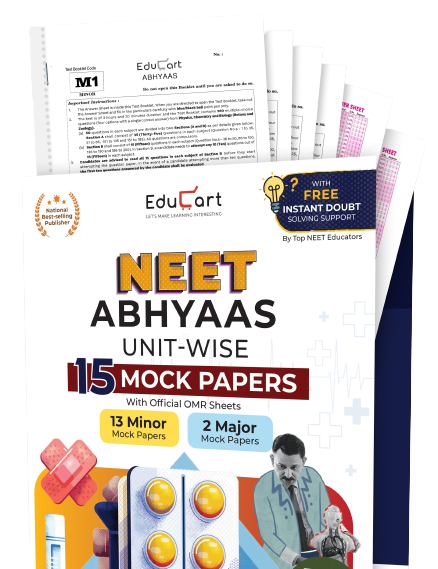# NCERT Books Class 9 MathematicsNCERT Books hold the most important role in Class 9 to 12 exam preparation. Every session begins with reading and understanding the concepts of the NCERT textbooks and exemplar books. Same is the case with Mathematics.

Below, we have provided direct download links to NCERT Books - Mathematics; both combined and separate chapter-wise PDFs.

Class 9 Mathematics Complete Book

Class 9 Mathematics Chapters

Chapter 1 Number Systems
Chapter 2 Polynomials
Chapter 3 Coordinate Geometry
Chapter 4 Linear Equations in One Variable
Chapter 5 Introduction to Euclid's Geometry
Chapter 6 Lines and Angles
Chapter 7 Triangles